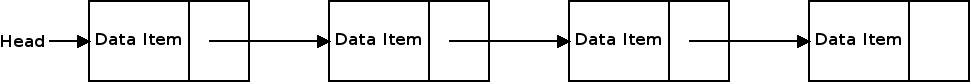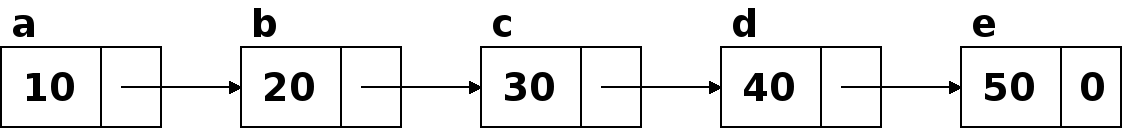### Motivation

So far we have seen the array data structure. Arrays are good for many tasks, but there are several array operations which are inefficient:

• Inserting into the beginning or middle.
• Removing an item from the beginning or middle.
• Adding to a full array.
• Expanding or shrinking the array.

Linked lists store a sequence of data, just like an array, but in a manner which makes the above operations more efficient.

A linked list is a fundamental data structure. The basic idea is that the list is composed of "nodes". Each node node contains one data element and the link to the next node in the list.Unlike arrays, the elements in a linked list are not stored in order in memory. In order to loop through the linked list, we must follow the links in each node to find the location of the next item in the list. In C++, the links are pointers. So each node in the list contains a pointer to the next node.

The first node in the linked list is called the "head". The location of the head must be stored so that we can find the rest of the list. The last element of the list is the one that doesn't contain a valid link to another node. In C++, we accomplish this by setting the pointer in the last node to NULL.

### Simple Example

The simplest way to create a linked list in C++ is using a struct. A struct is like a class, except it typically doesn't have private things or member functions, just data. This list will store ints, but we can use any type of data instead:


struct Node {
int data;
Node* next;
};


The struct is called "Node" and it represents one element in the list. Each Node contains the data, here a single int, and the link to the next Node. This is done using a pointer to a Node.

We can create a linked lists by creating a number of Node objects, and linking them together with the pointers. This example demonstrates creating a simple linked list in this manner. This program generates the following list:In order to loop through the list, we start with the first node, the head, and follow the links until we hit one with NULL for the next pointer.

How could we use this approach to write a function which prints a linked list?


void print(Node* head) {
Node* current = head;
while (current != NULL) {
cout << current->data << endl;
current = current->next;
}
}


### A Linked List Class

In the example above, we created a fixed number of nodes and linked them together. With linked lists, we almost always create the nodes dynamically with new. This allows the list to grow and shrink dynamically.

When using a dynamic linked list, it makes sense to separate it into a class. This way, we can contain the logic for managing the linked list in one place and reuse linked lists in our programs.

We can store the head node is a member variable in the class. The memory is allocated and de-allocated inside of the class.

How could we declare a linked list class to store a linked list of integers?


class IntList {
public:
IntList() {
}

void print() {
Node* current = head;
while (current != NULL) {
cout << current->data << endl;
current = current->next;
}
}

private:
struct Node {
int data;
Node* next;
};

};


We will also want a way to add new data to the list on the fly. With a linked list, it is easier to work with the front of the list because we have the head pointer.

How could we add a member function which takes an integer value and creates a new node for it at the start of the list?


void add(int value) {
Node* newNode = new Node;